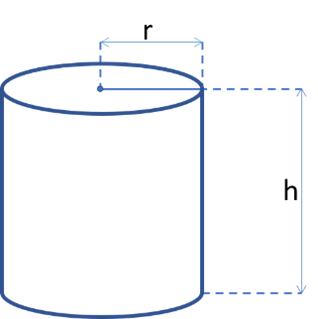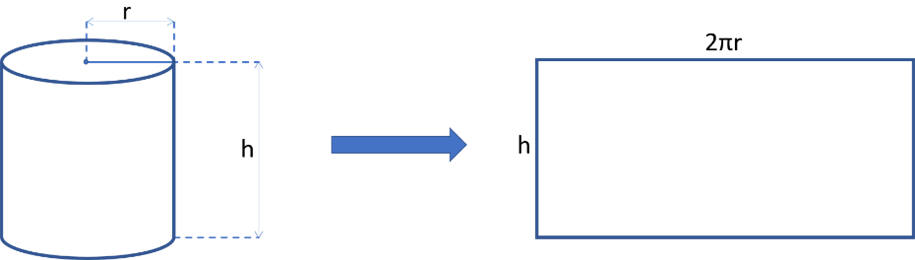Miscellaneous Calculators
Cylinder Volume Calculator

# Cylinder Volume Calculator

This calculator can be used as a cylinder volume calculator and surface area calculator. It also finds the lateral, base, and top surface areas.

Options

Height h = 5 m
Volume V = 141.37167
Lateral surface area L = 94.2477795
Top surface area T = 28.2743339
Base surface area B = 28.2743339
Total surface area A = 150.796447

There was an error with your calculation.This calculator finds the missing characteristics of a circular cylinder based on the known parameters. The parameters include cylinder height, radius, volume, lateral surface area, and total surface area. To find the missing characteristics, two of the parameters listed above must be known. Thus, the calculator can be used as a cylinder volume calculator and a cylinder surface area calculator.

## List of parametersThis calculator uses the following notation for the circular cylinder characteristics:

h – the height of the cylinder r – base radius V – volume L – lateral surface area A – total surface area

The additional characteristics used for the calculations are:

T – top surface area B – base surface area (B = T)

## Directions for use

To use the calculator, choose the calculation type from the drop-down menu on the top. The available options are:

• Calculate V, L, A | Given r, h
• Calculate h, L, A | Given r, V
• Calculate h, V, A | Given r, L
• Calculate r, V, A | Given h, L
• Calculate r, L, A | Given h, V

After choosing the type of calculation, enter the given values corresponding to the selected type.

For example, if you need to calculate the total area of a cylinder, lateral area of a cylinder, and cylinder volume, and if cylinder height and base radius are known (Calculate V, L, A | Given r, h), enter cylinder height, h, and base radius, r, into the corresponding fields.

Then you can choose the value of π used during the calculations. The default value is 3.1415926535898. Note that the default value will also be used if you enter a value very far from the actual value of π. For example, if you input π = 10, the value of 3.1415926535898 will be used during the calculations.

You can also choose the units (meters, centimeters, millimeters, miles, yards, feet, inches), and the number of significant figures (up to 9) for rounding the final answers.

After making all the choices, press "Calculate".

## Formulas

### Cylinder volume

The volume of a cylinder can be found by multiplying its base area by its height. The base of a circular cylinder is a circle with a radius r. The circle's surface area can be found as πr². Therefore, the volume of a cylinder, V, can be found with the following formula:

V = πr²h

### Lateral surface area

A cylinder's lateral surface area is occupied by its curved side. "Unrolling" the side surface of a cylinder onto a plane, we will get a rectangle, with one of the sides equal to h and the other side equal to the circumference of the base circle. The area of a rectangle can be found by multiplying the lengths of its sides. The circumference of the base circle can be found as 2πr. Therefore, the lateral surface area of a cylinder can be found with the following formula:

L = 2πrh### Base surface area (and top surface area)

A circular cylinder's top surface area, T, and the base surface area, B, are equivalent since the top and base are equal circles representing the bottom surfaces. B = T can be found with the circle surface area formula:

B = T = πr²

## The total surface area of a cylinder

A cylinder's total surface area is occupied by all its surfaces: top surface area, bottom surface area, and lateral surface area. Therefore, the total surface area of a cylinder, A, can be found as a sum of those surface areas:

A = B + T + L = πr² + πr² + 2πrh = 2πr² + 2πrh = 2πr(r + h)

## Calculation algorithms

Let's look at the algorithms the calculator uses for each calculation type.

Calculate V, L, A | Given r, h

In this case, the calculator will use the formulas presented above to find the missing cylinder characteristics.

Calculate h, L, A | Given r, V

The above formulas are based on a situation where h and r are known. Therefore, to use the above formulas, we always need to find h and r. In this situation, r is known, and we need to find h. Since the cylinder volume, V, is given, we can use the following formula to find h:

h = V / (πr²)

Now we know both h and r and can calculate the missing parameters.

Calculate h, V, A | Given r, L

r is known, and we need to find h, to be able to use the standard cylinder formulas. L is given. Therefore, h can be found as follows:

h = L / 2πr

Now we know both h and r and can calculate the missing parameters.

Calculate r, V, A | Given h, L

h is known, and we need to find r. L is given. Therefore, r can be found as follows:

r = L / 2πh

Now we know both h and r and can calculate the missing parameters.

Calculate r, L, A | Given h, V

h is known, and we need to find r. V is given. Therefore, r can be found as follows:

$$r=\sqrt{\frac{V}{πh}}$$

Now we know both h and r and can calculate the missing parameters.

## Real-life applications

Calculating various characteristics of a cylinder has many real-life applications. For example, knowing the surface area is necessary to determine the material needed to make a cylindrical container. The lateral area information is used when constructing plumbing pipes, and tubes for various purposes. Knowing a cylinder's volume is essential for estimating how much liquid or solid material can be stored in a cylindrical container.

### Example

What is the volume of a cylindrical water tank with a height of 5 meters and a base diameter of 4 meters?

Solution

To use the standard formula for cylinder volume, we need to know the height of the cylinder and its base radius. We are given the diameter of the base: d = 4 m. The base radius can be found with the following formula:

r = d/2 = 4/2 = 2

Now we have all the necessary parameters: h = 5, r = 2. Assuming that π = 3.14, the volume can be found as follows:

V = πr²h = 3.14 × (2)² × 5 = 3.14 × 4 × 5 = 62.8